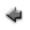previous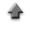home

# Multiple linear regression

The multiple linear regression model assumes that the statistical relationship between the response variable Yi(i=1,2,...,n) and the explanatory variables xij(i=1,2,...,n, j=1,2,...,k) is of the form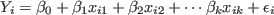,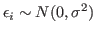,
where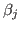(j=0,1,2,...,k) are the regression parameters and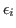denotes the independent normal random variables with zero mean and common variance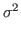.

• You can fit the multiple linear regression model,,to a data set.
• To start, click "Multiple linear regression" button on the application or select "Multiple linear regression" item from Tools in the menu bar.

If you use the sample data, click here, and save it in the appropriate directory (or folder).

You can fit a regression model having 3 parameters to the sample data.

1. Click "open data file" at the line "Data", and specify the data file prepared according to the above instruction.
2. When clicking the "Fit" button bellow, a summary of fitting is displayed.

## Data

1. Each label for data can be set in the first row in the data file. The label can not include spaces. Each label has to be delimited by space or tab.
2. Data must be inputted by normal width.
3. Data must be inputted in the data file according to the following instructions about the data errors.
• If the errors in the ordinate (y data) are unknown , the data must be inputted in order of x and y which are delimited by space or tab. Change the line, and input the data of the following row similarly until the input of the last data ends.
 x1 y1 x2 y2 . . . . . . xn yn
• If the errors in the ordinate are known, the data must be inputted in order of x, y and the error of y(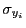) which are delimited by space or tab. Change the line, and input the data of the following row similarly until the input of the last data ends.
 x1 y1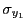x2 y2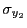. . . . . . . . . xn yn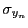4. In addition, you can use the table data form in the data file. You may include the column data in the data file which are not used to fit. In that case, the specification of the columns in the data file used to fit is needed at the line "Data columns" in the following "Input" instruction.

## Inputs

1. Click "Open data file", and specify the data file prepared according to the above instruction.
2. When the labels for data are used, check the "Data labels".
3. The columns in the data file to be used to fit a function model can be specified at the line "Data columns". The columns must be specified in order of the column of x, the column of y (and the column of the errors of y if the errors in ys are known) which are delimited by space. For instance, when x data are put at the third column, y data at the fifth column and the error of y at the sixth column in the data file, specify "3 5 6", and check the "Data columns" box.
4. Input the number of parameters at the line "Number of parameters" which must be delimited by space, and click the "Set" button.
5. Input the significance level at the line "Significance level" for the confidence intervals of the parameters and the tests of hypotheses about the regression model and click the "Set" button.

## Regression

1. A summary of the regression analysis is displayed when the "Fit" button is clicked.
2. When the "Show parameter estimates" button is clicked, the parameter estimates is displayed in another window.
3. When the "Show correlation coefficients" button is clicked, the correlation coefficients between the explanatory variables is displayed in another window.
4. When the "Residual plots" button is clicked, the plot of the studentized residuals againts the predicted values is displayed.
5. When the "Normal probability plots" button is clicked, the normal probability plots of the studentized residuals is displayed.
6. When the "Confidence intervals" button is clicked, the confidence intervals and the prediction intervals in the multiple linear regression is displayed in another window.

Kazushi Neichi